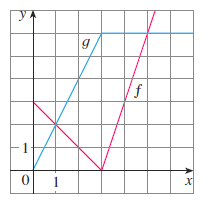Chapter 2.R, Problem 60E

Chapter
Section
Textbook Problem

# If f and g are the functions whose graphs are shown, let P ( x ) = f ( x ) g ( x ) , P ( x ) = f ( x ) g ( x ) , and C ( x ) = f ( g ( x ) ) .Find (a) P ' ( 2 ) ,(b) Q ' ( 2 ) , and (c) C ' ( 2 )(a)

To determine

To find: P'2

Explanation

By differentiating and using values from the graph

1) Formula:

i. Sum rule:

ddxfx+gx=ddxfx+ddxg(x)

ii. Difference rule:

ddxfx-gx=ddxfx-ddxg(x)

iii. Product rule:

ddxfx.gx=fxddxgx+gxddxfx

iv. Chain rule:

ddx(fgx=f'gx*g'(x)

2) Given:

Px=f

(b)

To determine

To find: Q'2

(c)

To determine

To find:C'(2)

### Still sussing out bartleby?

Check out a sample textbook solution.

See a sample solution

#### The Solution to Your Study Problems

Bartleby provides explanations to thousands of textbook problems written by our experts, many with advanced degrees!

Get Started

#### Convert the expressions in Exercises 8596 radical form. x1/3y3/2

Finite Mathematics and Applied Calculus (MindTap Course List)

#### 21. If the cost function for a commodity is graph the marginal cost function.

Mathematical Applications for the Management, Life, and Social Sciences

#### Rewritten in reverse order,

Study Guide for Stewart's Multivariable Calculus, 8th